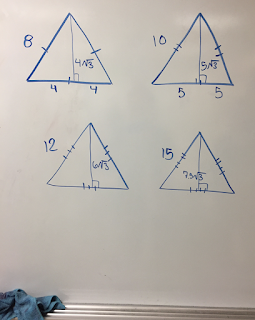Monday, January 29, 2018

Teaching Geometry - Special Right Triangles #VNPS

Today's lesson: Special Right Triangles - good for drawing and checking vocabulary

We were going to the boards to discover both special right triangles, using squares and equilateral triangles.

We started with 4 squares.  (I forgot to take square pictures). We had 4 different sizes and I asked them to find the diagonals.  Some started the Pythagorean Theorem and then they saw the pattern!

Then, we did 4 equilateral triangles:Then we drew altitudes.  I asked them to label their angles and find the altitude. What did they notice?I already taught the area of a regular hexagon using equilateral triangles and the Pythagorean Theorem, so they caught on to this one pretty quickly.Here is the google slide: Special Right Triangle Lesson

Here are the board problem: Special Right Triangle Board Problems.